# Looking for Relationships in the Data

When there are two series of data, there are a number of statistical measures that can be used to capture how the series move together over time.

## Correlations and Covariances

The two most widely used measures of how two variables move together (or do not) are the correlation and the covariance. For two data series, X (X1, X2,) and Y(Y, Y. . .), the covariance provides a measure of the degree to which they move together and is estimated by taking the product of the deviations from the mean for each variable in each period.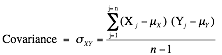The sign on the covariance indicates the type of relationship the two variables have. A positive sign indicates that they move together and a negative sign that they move in opposite directions. Although the covariance increases with the strength of the relationship, it is still relatively difficult to draw judgments on the strength of the relationship between two variables by looking at the covariance, because it is not standardized.

The correlation is the standardized measure of the relationship between two variables. It can be computed from the covariance :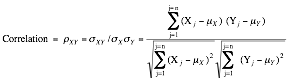The correlation can never be greater than one or less than negative one. A correlation close to zero indicates that the two variables are unrelated. A positive correlation indicates that the two variables move together, and the relationship is stronger as the correlation gets closer to one. A negative correlation indicates the two variables move in opposite directions, and that relationship gets stronger the as the correlation gets closer to negative one. Two variables that are perfectly positively correlated (rXY = 1) essentially move in perfect proportion in the same direction, whereas two variables that are perfectly negatively correlated move in perfect proportion in opposite directions.

## Regressions

A simple regression is an extension of the correlation/covariance concept. It attempts to explain one variable, the dependent variable, using the other variable, the independent variable.

### Scatter Plots and Regression Lines

Keeping with statistical tradition, let Y be the dependent variable and X be the independent variable. If the two variables are plotted against each other with each pair of observations representing a point on the graph, you have a scatterplot, with Y on the vertical axis and X on the horizontal axis.  Figure A1.3 illustrates a scatter plot.

Figure A1.3: Scatter Plot of Y versus XIn a regression, we attempt to fit a straight line through the points that best fits the data. In its simplest form, this is accomplished by finding a line that minimizes the sum of the squared deviations of the points from the line. Consequently, it is called an ordinary least squares (OLS) regression. When such a line is fit, two parameters emerge—one is the point at which the line cuts through the Y-axis, called the intercept of the regression, and the other is the slope of the regression line:

Y = a + bX

The slope (b) of the regression measures both the direction and the magnitude of the relationship between the dependent variable (Y) and the independent variable (X). When the two variables are positively correlated, the slope will also be positive, whereas when the two variables are negatively correlated, the slope will be negative. The magnitude of the slope of the regression can be read as follows: For every unit increase in the dependent variable (X), the independent variable will change by b (slope).

### Estimating Regression Parameters

Although there are statistical packages that allow us to input data and get the regression parameters as output, it is worth looking at how they are estimated in the first place. The slope of the regression line is a logical extension of the covariance concept introduced in the last section. In fact, the slope is estimated using the covariance:The intercept (a) of the regression can be read in a number of ways. One interpretation is that it is the value that Y will have when X is zero. Another is more straightforward and is based on how it is calculated. It is the difference between the average value of Y, and the slope-adjusted value of X.Regression parameters are always estimated with some error or statistical noise, partly because the relationship between the variables is not perfect and partly because we estimate them from samples of data. This noise is captured in a couple of statistics. One is the R2 of the regression, which measures the proportion of the variability in the dependent variable (Y) that is explained by the independent variable (X). It is also a direct function of the correlation between the variables:An R2 value close to one indicates a strong relationship between the two variables, though the relationship may be either positive or negative. Another measure of noise in a regression is the standard error, which measures the “spread” around each of the two parameters estimated—the intercept and the slope. Each parameter has an associated standard error, which is calculated from the data:

Standard Error of Intercept = SEa =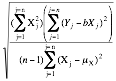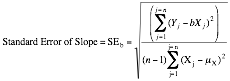If we make the additional assumption that the intercept and slope estimates are normally distributed, the parameter estimate and the standard error can be combined to get a t-statistic that measures whether the relationship is statistically significant.

t-Statistic for Intercept = a/SEa

t-Statistic from Slope = b/SEb

For samples with more than 120 observations, a t-statistic greater than 1.95 indicates that the variable is significantly different from zero with 95% certainty, whereas a statistic greater than 2.33 indicates the same with 99% certainty. For smaller samples, the t-statistic has to be larger to have statistical significance.

### Using Regressions

Although regressions mirror correlation coefficients and covariances in showing the strength of the relationship between two variables, they also serve another useful purpose. The regression equation described in the last section can be used to estimate predicted values for the dependent variable, based on assumed or actual values for the independent variable. In other words, for any given Y, we can estimate what X should be:

X = a + b(Y)

How good are these predictions? That will depend entirely on the strength of the relationship measured in the regression. When the independent variable explains a high proportion of the variation in the dependent variable (R2 is high), the predictions will be precise. When the R2 is low, the predictions will have a much wider range.

### From Simple to Multiple Regressions

The regression that measures the relationship between two variables becomes a multiple regression when it is extended to include more than one independent variables (X1, X2, X3, X4 . . .) in trying to explain the dependent variable Y. Although the graphical presentation becomes more difficult, the multiple regression yields output that is an extension of the simple regression.

Y = a + bX1 + cX2 + dX3 + eX4

The R2 still measures the strength of the relationship, but an additional R2 statistic called the adjusted R2 is computed to counter the bias that will induce the R2 to keep increasing as more independent variables are added to the regression. If there are k independent variables in the regression, the adjusted R2 is computed as follows: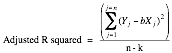Multiple regressions are powerful tools that allow us to examine the determinants of any variable.

### Regression Assumptions and Constraints

Both the simple and multiple regressions described in this section also assume linear relationships between the dependent and independent variables. If the relationship is not linear, we have two choices. One is to transform the variables by taking the square, square root, or natural log (for example) of the values and hope that the relationship between the transformed variables is more linear. The other is to run nonlinear regressions that attempt to fit a curve (rather than a straight line)  through the data.

There are implicit statistical assumptions behind every multiple regression that we ignore at our own peril. For the coefficients on the individual independent variables to make sense, the independent variable needs to be uncorrelated with each other, a condition that is often difficult to meet. When independent variables are correlated with each other, the statistical hazard that is created is called multicollinearity. In its presence, the coefficients on independent variables can take on unexpected signs (positive instead of negative, for instance) and unpredictable values. There are simple diagnostic statistics that allow us to measure how far the data may be deviating from our ideal.

The actual values that t-statistics need to take can be found in a table for the t distribution, which can be found in any standard statistics book or software package.